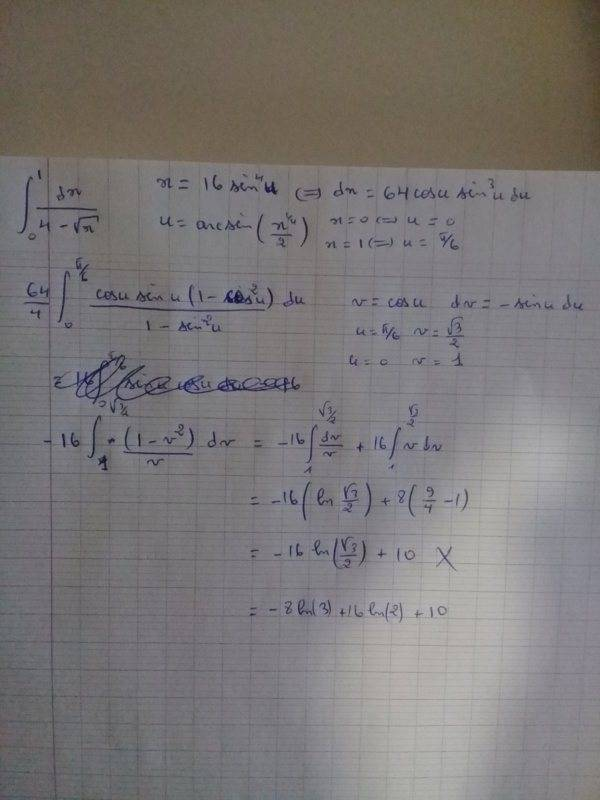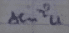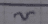# Trigonometric definite integral of 1/(4-sqrt(x))

• archaic
C$$I have that ##x=16\sin^4u \Leftrightarrow u=\arcsin\frac{\sqrt x}{2}##, and ##v=\cos u=\cos\left(\arcsin\frac{\sqrt x}{2}\right)## [which I have calculated wrongly as ##\frac{4-\sqrt x}{2}## and just figured it out writing this response, thank you very much!].And nicely typeset in ##\LaTeXf #### archaic Homework Statement$$\int_0^1\frac{dx}{4-\sqrt x}$$Relevant Equations N/A This could be solved by the substitution ##u=\sqrt x##, but I wanted to do it using a trigonometric one. The answer is false, but I don't see the wrong step. Thank you for your time! [Poster has been reminded to learn to post their work using LaTeX]Last edited by a moderator: Challenge to decipher someones photographed scribbles. ##\LaTeX## is soooo much more legible. Do I discern a ##\ \ 1-\sin^2u\quad## () in the denominator and on the next line a simple ##v## ()? Challenge to decipher someones photographed scribbles. ##\LaTeX## is soooo much more legible. Do I discern a ##\ \ 1-\sin^2u\quad## ( View attachment 256961 ) in the denominator and on the next line a simple ##v## ( View attachment 256962 )? I mad the change of variable ##v=\cos u##, changed the sine to cosine squared and canceled one power, since there is another cosine in the nominator. I am sorry for not formatting the math, but it would've been a bit tedious (more than having to decipher someone else's scribbles. thank you very much!). My bad I missed that one. Let me try another: how much is$$16 \int_1^\sqrt{3\over 4} v\, dv$$My bad I missed that one. Let me try another: how much is$$16 \int_1^\sqrt{3\over 4} v\, dv$$Oh, I have doubly squared the ##3##, thank you. My original concern was about the indefinite integral, though, as that manipulation is getting me a wrong one.$$-16\int \frac{dv}{v}+16\int v\,dv=-16\ln|v|+8v^2+C
I have that ##x=16\sin^4u \Leftrightarrow u=\arcsin\frac{\sqrt x}{2}##, and ##v=\cos u=\cos\left(\arcsin\frac{\sqrt x}{2}\right)## [which I have calculated wrongly as ##\frac{4-\sqrt x}{2}## and just figured it out writing this response, thank you very much!].

•BvU
And nicely typeset in ##\LaTeX## too !

My original concern was about the indefinite integral
Intriguing. Needs checking because we move into the complex range. Haven't got it sorted out, but I do notice a difference between the original and the one you derived from it.

And nicely typeset in ##\LaTeX## too !

Intriguing. Needs checking because we move into the complex range. Haven't got it sorted out, but I do notice a difference between the original and the one you derived from it.
How it is in the picture is correct, I meant that I have made a mistake when switching back to ##x##.